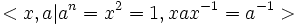# Dihedral group

WARNING: POTENTIAL TERMINOLOGICAL CONFUSION: Please don't confuse this with dicyclic group (also called binary dihedral group)
This article defines a group property: a property that can be evaluated to true/false for any given group, invariant under isomorphism
View a complete list of group properties
VIEW RELATED: Group property implications | Group property non-implications |Group metaproperty satisfactions | Group metaproperty dissatisfactions | Group property satisfactions | Group property dissatisfactions

This is a family of groups parametrized by the natural numbers, viz, for each natural number, there is a unique group (upto isomorphism) in the family corresponding to the natural number. The natural number is termed the parameter for the group family

The dihedral group with parameter$n$, denoted sometimes as$D_n$ and sometimes as$D_{2n}$ is defined in the following equivalent ways:$$
• It is the group of symmetries of a regular$n$-gon in the plane, viz the plane isometries that preserves the set of points of the regular$n$-gon.